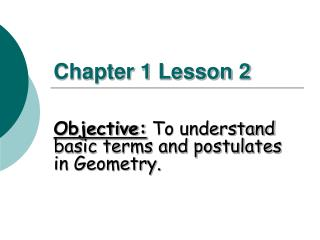DownloadDownload PresentationChapter 1 Lesson 2

# Chapter 1 Lesson 2

Download Presentation## Chapter 1 Lesson 2

- - - - - - - - - - - - - - - - - - - - - - - - - - - E N D - - - - - - - - - - - - - - - - - - - - - - - - - - -
##### Presentation Transcript

1. Chapter 1 Lesson 2 Objective: To understand basic terms and postulates in Geometry.

2. Points • Points are a location that do not have actual size. • How to Sketch: Using dots • How to label: Use capital letters • Never name two points with the same letter (in the same sketch). Space is defined as the set of all points. A B A C

3. Lines extend indefinitely and have no thickness or width. How to sketch : using arrows at both ends. How to name: 2 ways (1) small script letter – line n (2) any two points on the line - Never name a line using three points - Lines n A B C

4. Postulates and Axioms Postulate or Axiom is an accepted statement or fact. Postulate 1-1                                                                              Through any two points there is exactly one line. Line t is the only line that passes through points A and B.

5. Collinear Points • Collinear points are points that lie on the same line. (The line does not have to be visible.) • A point lies on the line if the coordinates of the point satisfy the equation of the line. C A B C A B Collinear Non collinear

6. Example 1: Collinear Points To find if A (1, 0) is collinear with the points on the line y = -3x + 3. Substitute x = 1 and y = 0 in the equation. 0 = -3 (1) + 3 0 = -3 + 3 0 = 0 The point A satisfies the equation, therefore the point is collinear with the points on the line.

7. Planes • A plane is a flat surface that extends indefinitely in all directions. • How to sketch: Use a parallelogram (four sided figure) • How to name: 2 ways (1) Capital script letter – Plane M (2) Any 3 non collinear points in the plane - Plane: ABC/ ACB / BAC / BCA / CAB / CBA A M B C Other Horizontal Plane Vertical Plane

8. Example 2: Planes Different planes in a figure: Plane ABCD Plane EFGH Plane BCGF Plane ADHE Plane ABFE Plane CDHG A B D C E F H G

9. Example 3: Planes Other planes in the same figure: Any three non collinear points determine a plane. Plane AFGD Plane ACGE

10. Example 4: Coplanar Coplanar Objects Coplanar objects (points, lines, etc.) are objects that lie on the same plane. The plane does not have to be visible. Are the following points coplanar? A, B, C ? Yes A, B, C, F ? No H, G, F, E ? Yes E, H, C, B ? Yes A, G, F ? Yes C, B, F, H ? No

11. Intersection of Figures (Postulate 1-2) The intersection of two figures is the set of points that are common in both figures. The intersection of two lines is a point. m Line m and line n intersect at point P. P n

12. 2 Possibilities of Intersection of a Line and a Plane (1) Line passes through plane – intersection is a point. (2) Line lies on the plane - intersection is a line.

13. Intersection of Two Planes is a Line (Postulate 1-3) B P A R Plane P and Plane R intersect at the line

14. Assignment Pages 13-16 #1-58 even